Foxtable(狐表)用户栏目专家坐堂 → 查找是否存在行 代码运行错误 无法运行下去

共有310人关注过本帖树形打印复制链接

# 主题：查找是否存在行 代码运行错误 无法运行下去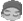1楼 | 信息 | 搜索 | 邮箱 | 主页 | UC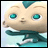加好友发短信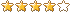代码运行错误  麻烦老师看看  谢谢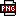此主题相关图片如下：微信图片_20200116203856.png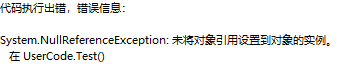代码如下  ：If Tables("人员关联表").Current("人员类型")<>"员额检察官"  Then    Dim ab As String =Tables("人员关联表").Current("姓名")    Dim ab1 As String =Tables("人员关联表").Current("部门受案号")    MessageBox.Show(ab)    MessageBox.Show(ab1)    Dim dr11 As DataRow = DataTables("告知内容").sqlFind("姓名= '" & ab & "'And 部门受案号 = '" & ab1 & "'")    MessageBox.Show(dr11("姓名"))    MessageBox.Show(dr11("部门受案号"))    If dr11 Is Nothing Then '如果不存在同编号的订单        MessageBox.Show("哈哈")        Tables("告知内容").addnew        Tables("告知内容").Current("姓名")=Tables("人员关联表").Current("姓名")        Tables("告知内容").Current("人员类型")="案件关联人"        Tables("告知内容").Current("部门受案号")=Tables("人员关联表").Current("部门受案号")        Tables("告知内容").Current("操作单位")=Tables("人员关联表").Current("操作单位")        Tables("告知内容").save    End IfEnd If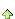2楼 | 信息 | 搜索 | 邮箱 | 主页 | UC加好友发短信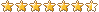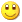Post By：2020/1/17 9:13:00 [只看该作者]

 先判断再使用！先判断再使用！先判断再使用！dim r2 as row =  Tables("人员关联表").CurrentIf r2 isnot nothing andalso r2("人员类型")<>"员额检察官"  Then    Dim ab As String =r2("姓名")    Dim ab1 As String =r2("部门受案号")    MessageBox.Show(ab)    MessageBox.Show(ab1)    Dim dr11 As DataRow = DataTables("告知内容").sqlFind("姓名= '" & ab & "'And 部门受案号 = '" & ab1 & "'")    If dr11 Is Nothing Then '如果不存在同编号的订单MessageBox.Show(dr11("姓名"))MessageBox.Show(dr11("部门受案号"))        MessageBox.Show("哈哈")        dim r as row = Tables("告知内容").addnew        r("姓名")=r2("姓名")        r("人员类型")="案件关联人"        r("部门受案号")=r2("部门受案号")        r("操作单位")=r2("操作单位")        Tables("告知内容").save    End IfEnd If